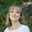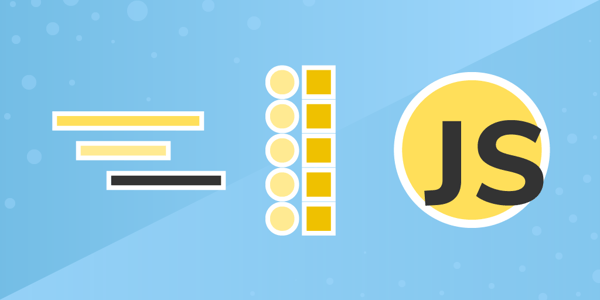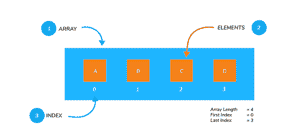# JavaScript Array Methods: how to use map and reduce

Nov 23, 2020 - 8 min readAmanda FawcettMethods are property names that we can assign to a functions. They can be invoked at any time just by using the name to execute a function. Array objects also have methods and properties that allow us modify or query them onto the object.

In JavaScript, array methods make it easy to manage and organize data in a convenient way. Today, we will be diving into two popular array methods: map() and reduce. These methods provide a modified version of an array and make arrays even more convenient.

Today, we will learn:

## JavaScript arrays refresher

When creating variables in JavaScript, it can be tricky to access a range of values. Similarly, creating objects requires you to create key names for all the data, which can lead to clutter. Arrays are the solution. This data structure manages ordered data in one interface.

Arrays are a collection of individual values separated by a comma, each with its own index/location. Arrays are objects with methods and properties used for managing data items in an orderly fashion.

They are list-like data structures that group data together, making them accessible through numerical indices.In JavaScript, arrays are easy to declare. Below, we create an array of fruit types and store them in an orderly fashion.

var fruit = [
"Orange",
"Apple",
"Banana"
];


Arrays in JavaScript have special features that make them particularly useful, including:

• They are dynamic
• They can be sparse or dense
• They are mutable
• They have methods and properties to make organization convenient

## What are array methods?

Array objects have methods and properties that allows us to modify or query them onto an object. These make it much easier to manage, access, and organize data. This way, we don’t have to create custom objects.

The property of an array is an attribute of that array, such as length or memory size. They are usually static values that can be used to change a particular quality of the object. prototype and length are common properties.

Methods of an array are actions that we can apply to an array. These are similar to properties but are of the type function. push() and pop() are common array methods that allow us to add or remove elements.

Refresher: Functions are a set of instructions that carry out a task. They allow us to reuse code anywhere in the program.

Array methods help make your programs far more convenient and useful. Next, we’ll look at two unique JavaScript array methods that make arrays even more convenient: map() and reduce.

## JavaScript map() method

The map() method is used to get a modified version of the array or a reduced value using callback functions. map() applies a function to each array element and creates a new array of the returned values.

The syntax of the method is as follows:

array.map(function(currentValue, index, arr), thisValue)


The map() method accepts two parameters:

• function(currentValue, index, arr): This is a required parameter that runs on each element of array. It contains three parameters: currentValue, index and arr.
• thisValue: This parameter is optional. It holds the value of passed to the function.

The map method will take a function invoked for each element in the array as in input. The function that is be passed is given arguments by the map method in the following order.

function callbackfn(value: any, index: number, array: any[])


For each element, the callbackfn will be passed with the value of the element as the first argument, followed by the index of the element as the second argument. Lastly the array itself as the third argument. This callbackfn function takes between 0 to 3 arguments.

Finally, a new array with all the returned values from the callbackfn function will be returned by the map method. Check out an example below.

var arr = [10, 20, 30, 40, 50]; // initialise an array and assign to arr
var arr1 = arr.map(a => a * 2); // double the element in the array
console.log("arr:",arr); // print original array
console.log("doubled array:",arr1); // print doubled array 

Note: You can see that the map method maps the arrow function to each element and returns a new array. The original array remains unchanged.

## map() examples and uses

There are many uses of map() in your JavaScript code.Let’s break down the most common ones.

### Generic use of map()

Below, our code shows how to use this method on a String to generate an array of bytes in ASCII encoding.

let map = Array.prototype.map
let a = map.call('Hello World', function(x) {
return x.charCodeAt(0)
})

### Mapping an array of numbers to an array of their square roots

Below, our code takes an array of numbers and creates a new array with the square roots of each number.

let numbers = [3, 25, 100]
let roots = numbers.map(function(num) {
return Math.sqrt(num)
})

### Mapping an array of numbers with a function containing an argument

Below, our code shows how map() can be used alongside a a function that has one argument. The argument will be assigned automatically from each element of the array.

let numbers = [3, 25, 100]
let doubles = numbers.map(function(num) {
return num * 2
})

Note: Since map() builds a new array, you should not use this method if:

• You are not using the array that is returned
• You are not returning any value from the callback

## Keep the learning going.

Learn how to build with JavaScript without scrubbing through videos or documentation. Educative’s text-based courses are easy to skim and feature live coding environments, making learning quick and efficient.

JavaScript in Detail: From Beginner to Advanced

## JavaScript reduce method

The reduce method reduces the array to a single value from left to right. This method leaves the original array unchanged.

The syntax of the method is as follows:

arr.reduce(<function>);


The reduce method takes a function invoked for each element in the array. It uses the reduced value of the previous element to the next. The reduce method gives arguments to the passed function in the following order:

function callbackfn(prev: any, curr: any, index: number, array: number[])


For each element, the callbackfn will be passed with the previous callbackfn function’s return value as the first argument, and the value of the element as the second argument.

This is followed by the index of the element as the third argument. Lastly, the array itself is taken as the fourth argument.

The callbackfn function returns a value passed onto the callbackfn function for the next element. If the array has only one value, that value is returned. For an empty array, an error is thrown.

Let’s learn more about reduce with an example below.

var arr = [10, 20, 30, 40, 50]; // initialise an array and assign to arr
var val = arr.reduce((prev, curr) => prev + curr); // reduce element to sum
console.log("arr:",arr); // print original array
console.log("reduced val:",val); // print element returned by reduce 

We can see here that the arrow function takes the previous value prev and adds it to the value iterated in the array curr. The reduce method sums the entire array.

Note: We can use the reduceRight method to apply the reduce method in the opposite direction.

## reduce examples and uses

There are many uses of reduce in your JavaScript code.Let’s break down the most common ones.

### Sum the values of an array

We can use reduce to sum all the values of an array in an easy way.

let sum = [0, 1, 2, 3].reduce(function (accumulator, currentValue) {
return accumulator + currentValue
}, 0)

### Flatten an array of arrays

let flattened = [[0, 1], [2, 3], [4, 5]].reduce(
function(accumulator, currentValue) {
return accumulator.concat(currentValue)
},
[]
)

### Group objects by a property

let people = [
{ name: 'Matt', age: 25 },
{ name: 'Asma', age: 23 },
{ name: 'Cami', age: 29 }
];

function groupBy(objectArray, property) {
return objectArray.reduce(function (acc, obj) {
let key = obj[property]
if (!acc[key]) {
acc[key] = []
}
acc[key].push(obj)
return acc
}, {})
}

let groupedPeople = groupBy(people, 'age')

## Using reduce and map() together

Now let’s learn how an example of how we can use the two methods together to make certain tasks easier. Often, we need to count array elements that satisfy a certain condition. Here’s how it’s done:

1. Map the array into an array of zeros and ones.
2. Reduce the array of zeros and ones into the sum.

The final output is a count of elements that satisfy a given condition.

var arr = ['Hello', 1, true, NaN, 'Bye']; // initialise an array of elements
var countArr = arr.map(ele => typeof ele === 'string' ? 1 : 0); // map to 0 and 1
var sum = countArr.reduce((prev, curr)=> prev + curr); // reduce for sum
console.log("arr:",arr); // print original array
console.log("array from map:", countArr); // print array returned from map method
console.log("number of Strings:",sum); // print number of strings 

On line 2, we apply map to get an array of ones and zeroes. Each satisfying element has one value. In line 3, we use reduce to find the sum of the array. This means we have a count of ones. then, using combo, we find the element count in the array assigned to arr where the element is a string.

Note: We can also use these methods together to find the number of elements in a two-dimensional array.

## What to learn next

Congrats! You’ve now taken a deep dive into JavaScript’s map() and reduce array methods. These will improve your code a lot. There’s still more to learn when it comes to array methods. Next, you should check out the following:

• some()
• every()
• flat()
• filter()
• forEach()
• flatMap()

To get started with these array methods and get some practice with map() and reduce, check out Educative’s course JavaScript in Detail: From Beginner to Advanced. In this project-based course you will dissect every part of JavaScript from beginning to advanced. You will be tasked with four hands-on projects and formal tests to solidifying your learning. By the end, you’ll be a proficient JavaScript developer.

Happy learning!